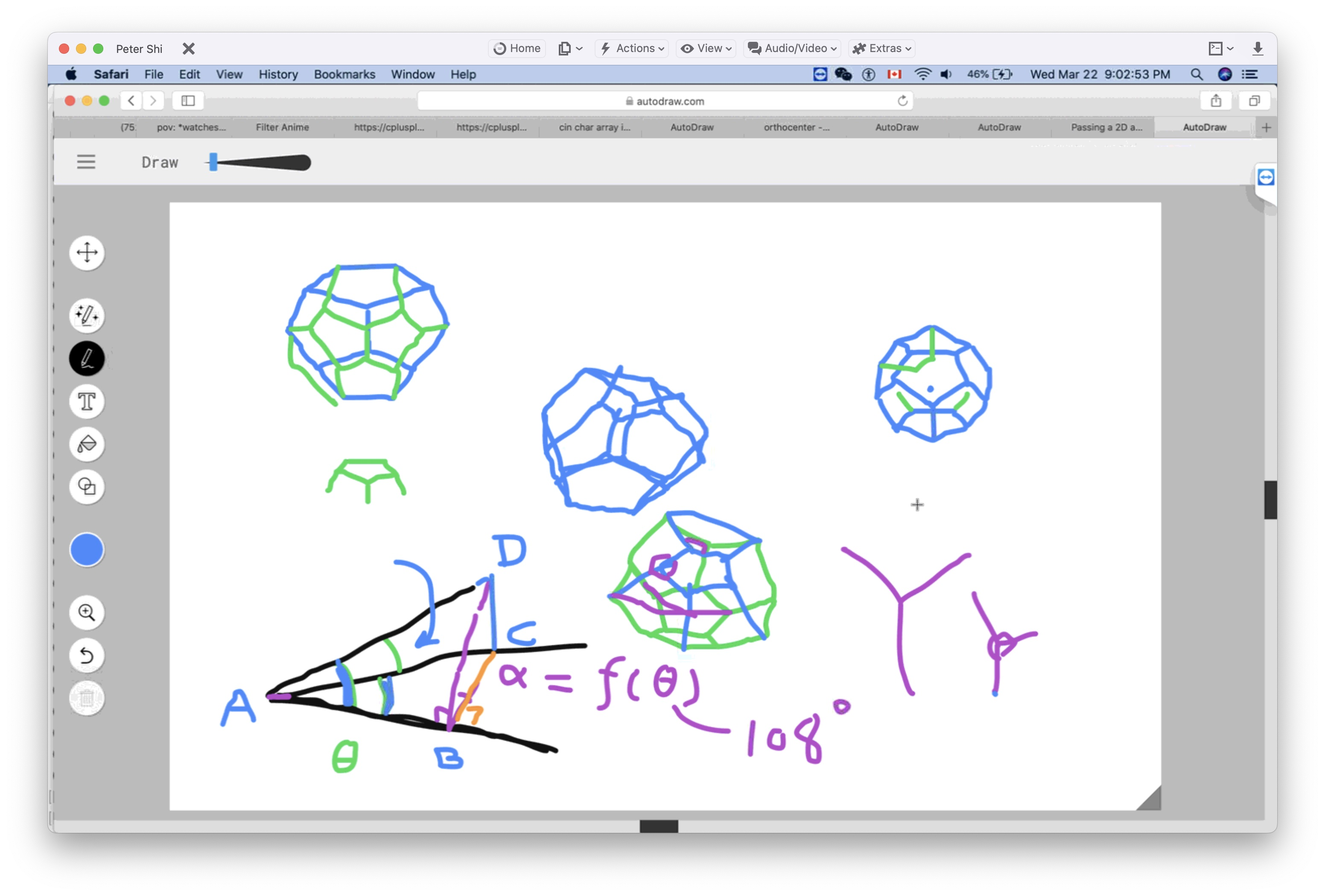This is what it looks like when finished: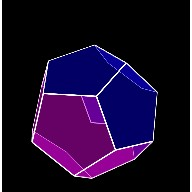First we want to calculate the angle between any 2 adjacent ⬠ faces, β in the above diagram. There is a generic relation between β and ⍺. In our specific case of dodecahedron ⍺ = 108º. So β = 116.7º.

draw the bottom ⬠ :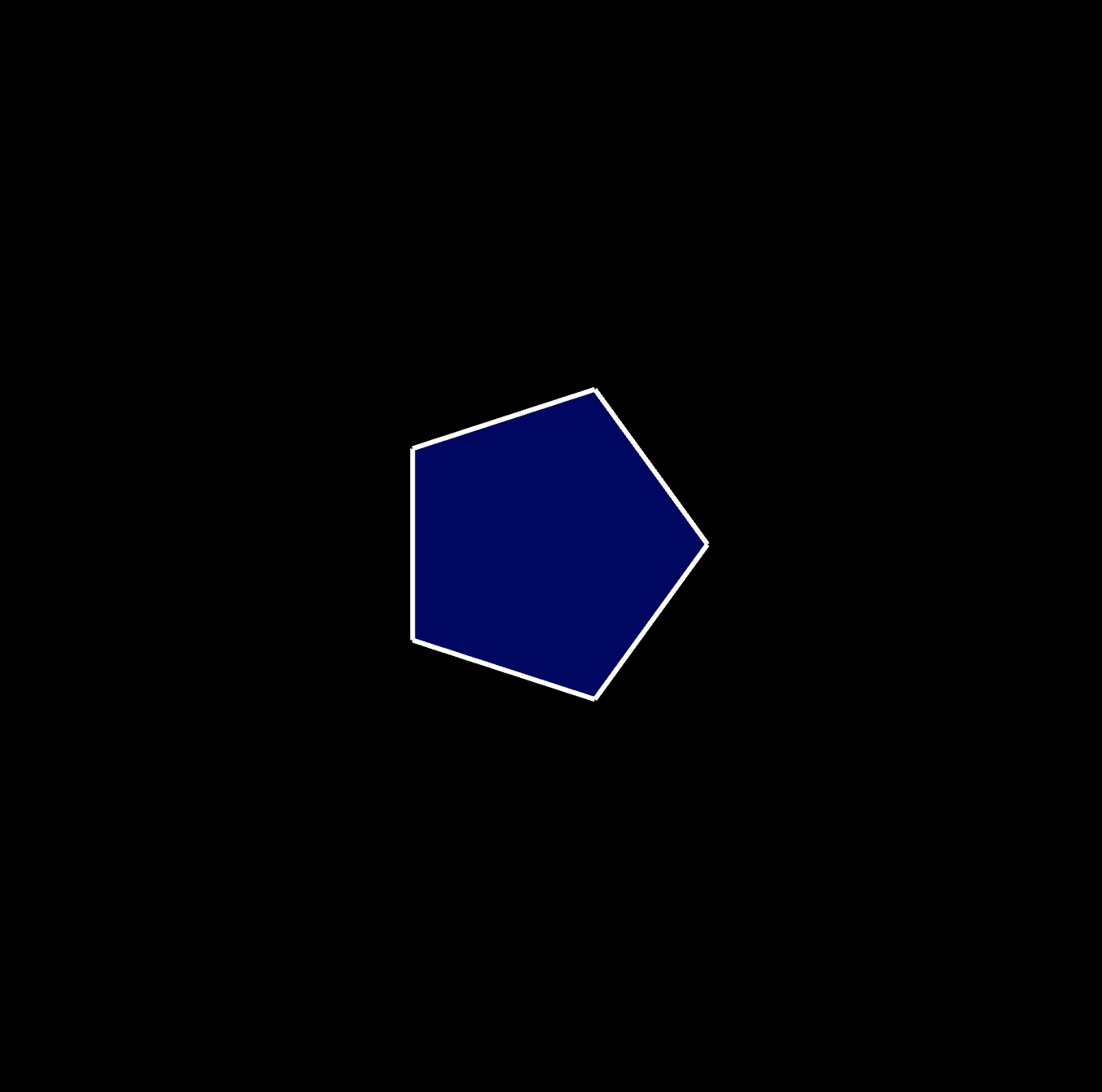the 1st ⬠ adjacent to the bottom ⬠ :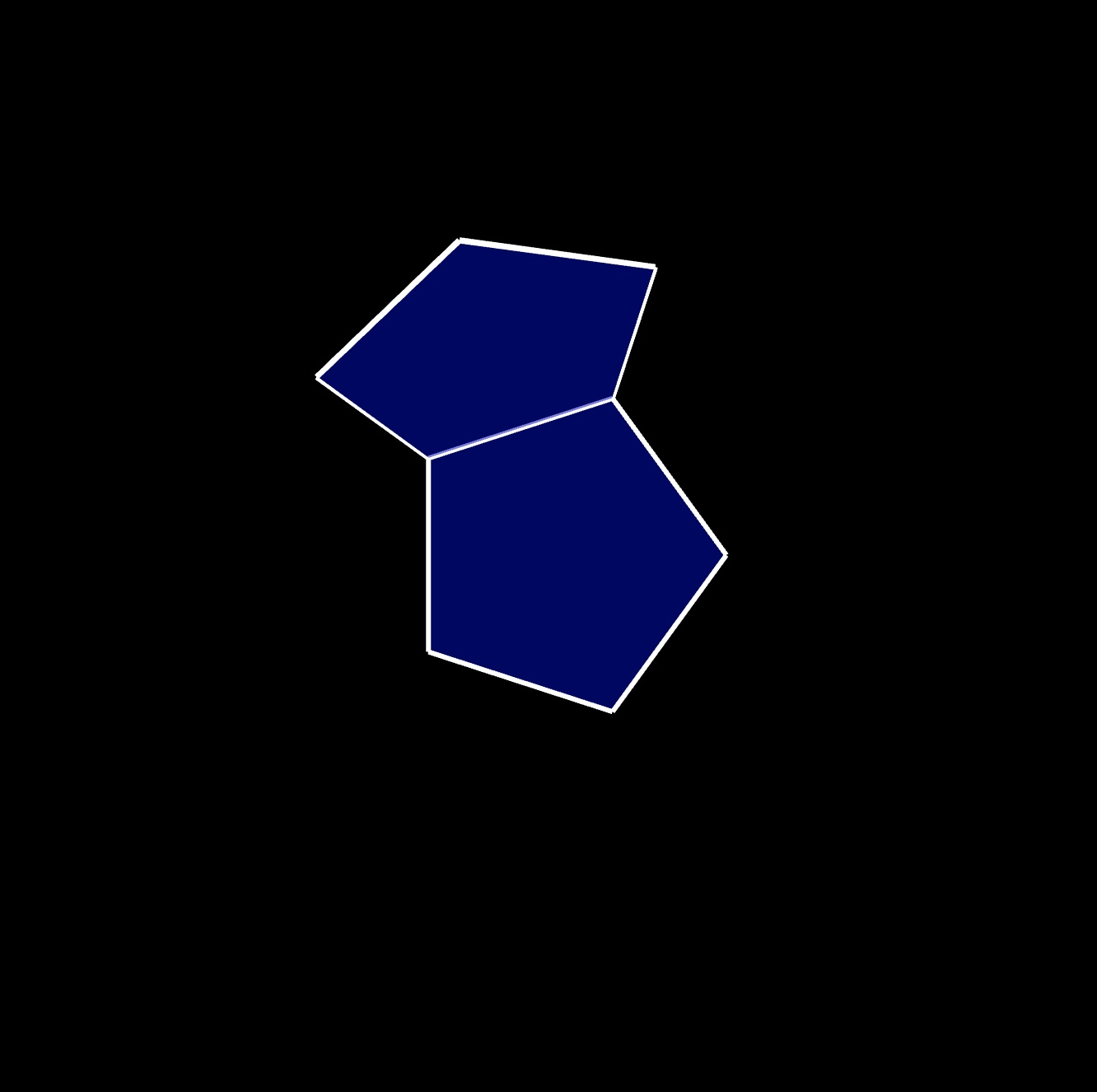the 2nd ⬠ adjacent to the bottom ⬠ :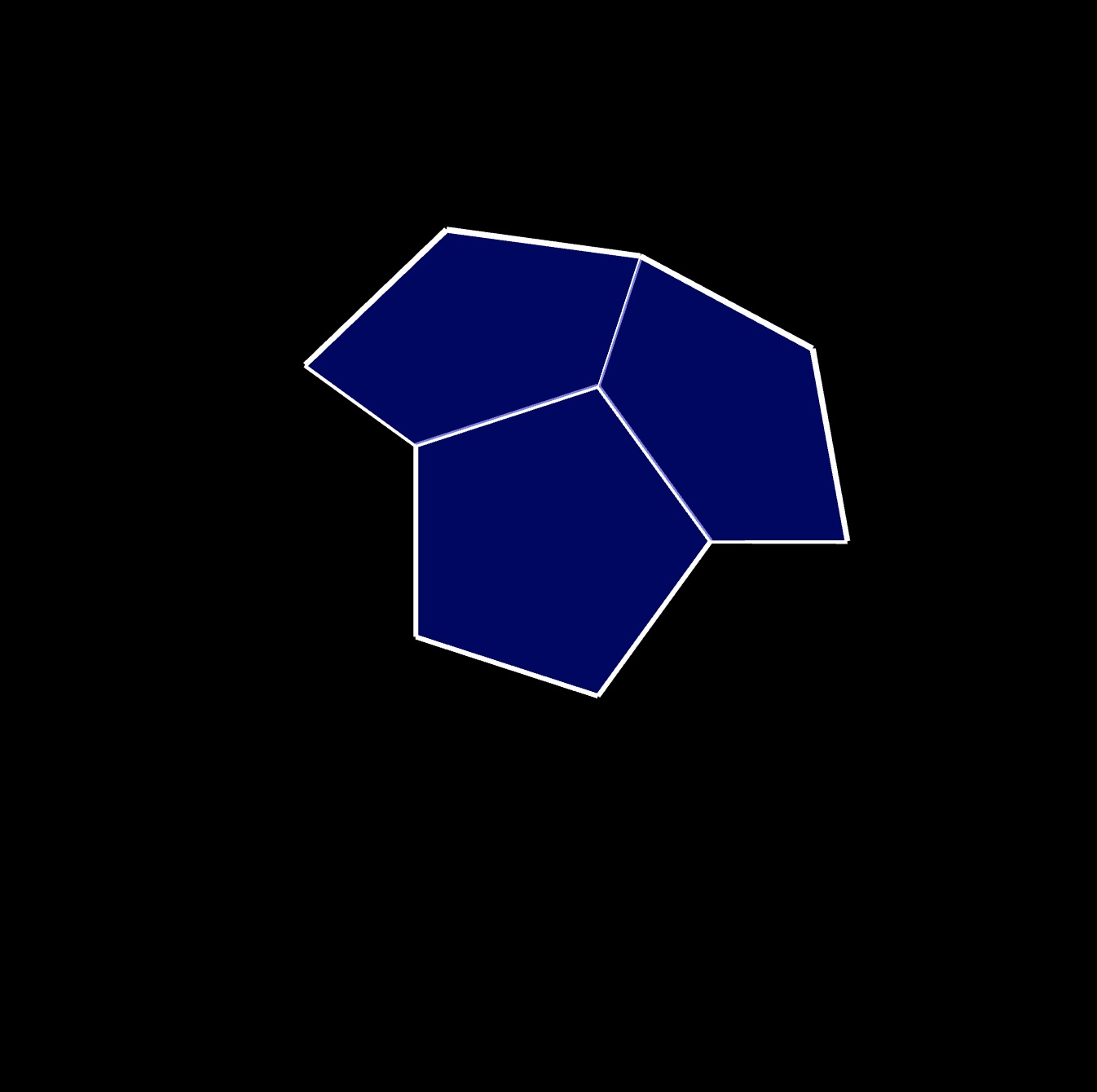the 3rd ⬠ adjacent to the bottom ⬠ :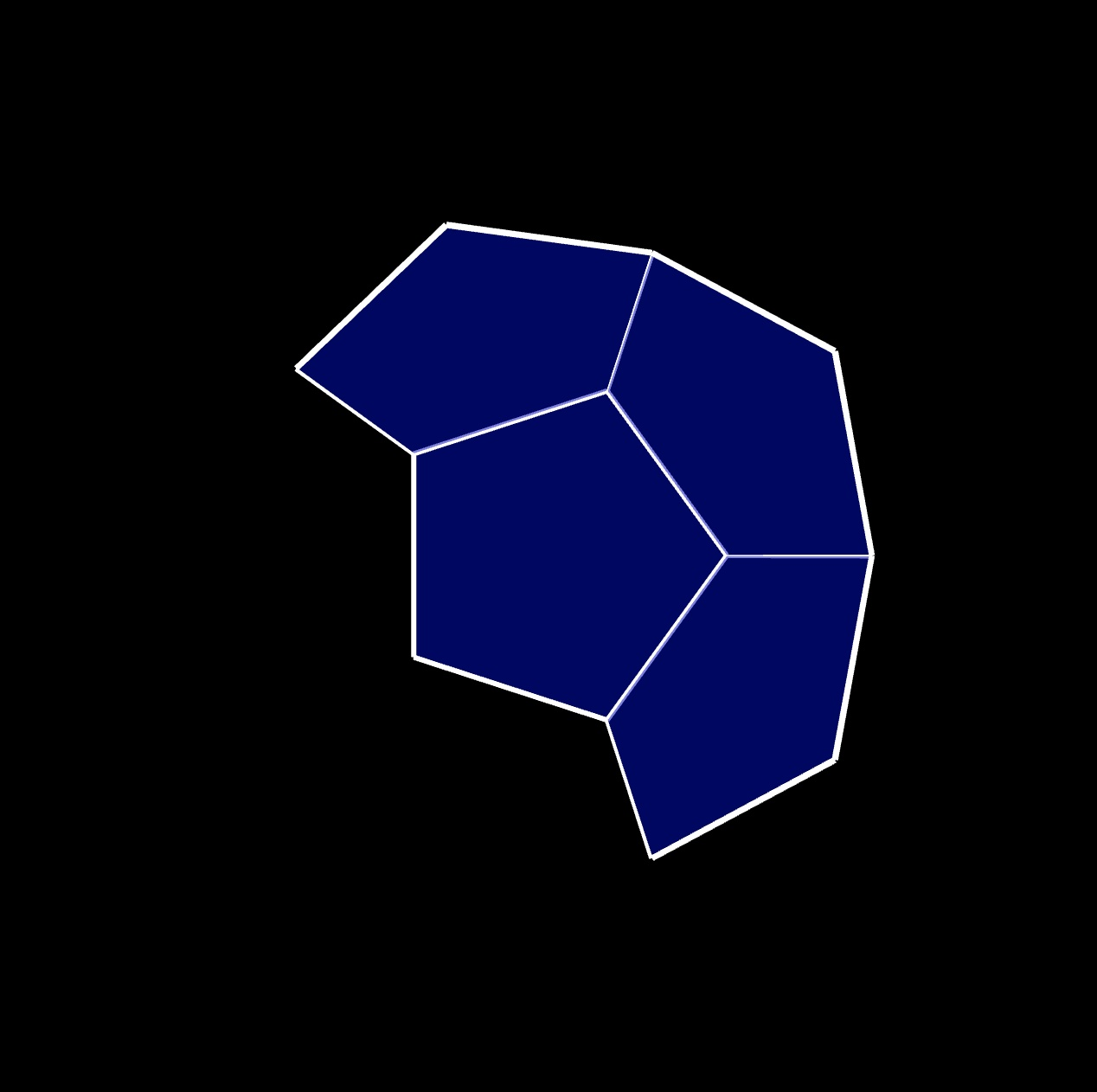draw the 4th ⬠ adjacent to the bottom ⬠ :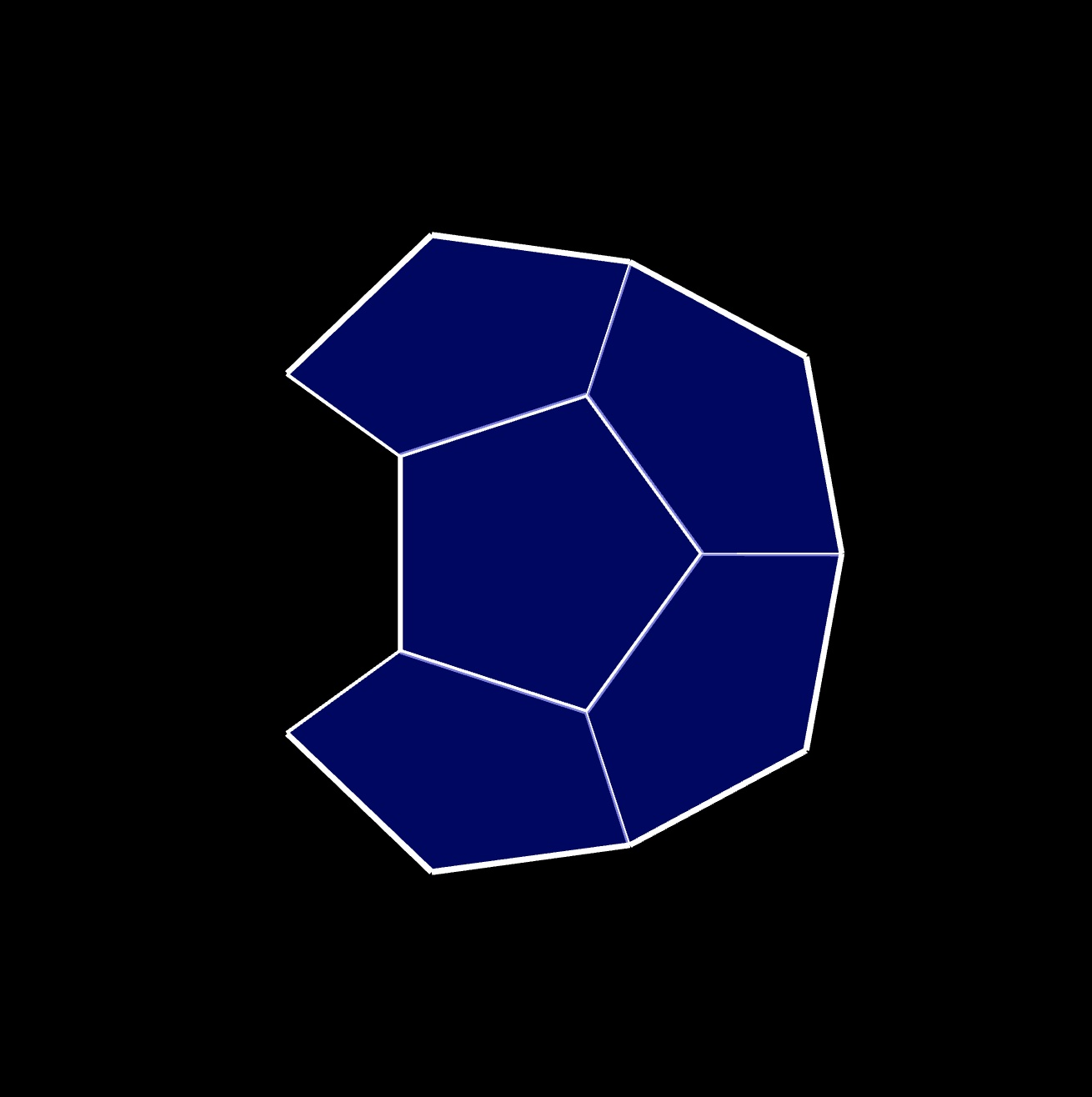the last ⬠ adjacent to the bottom ⬠ :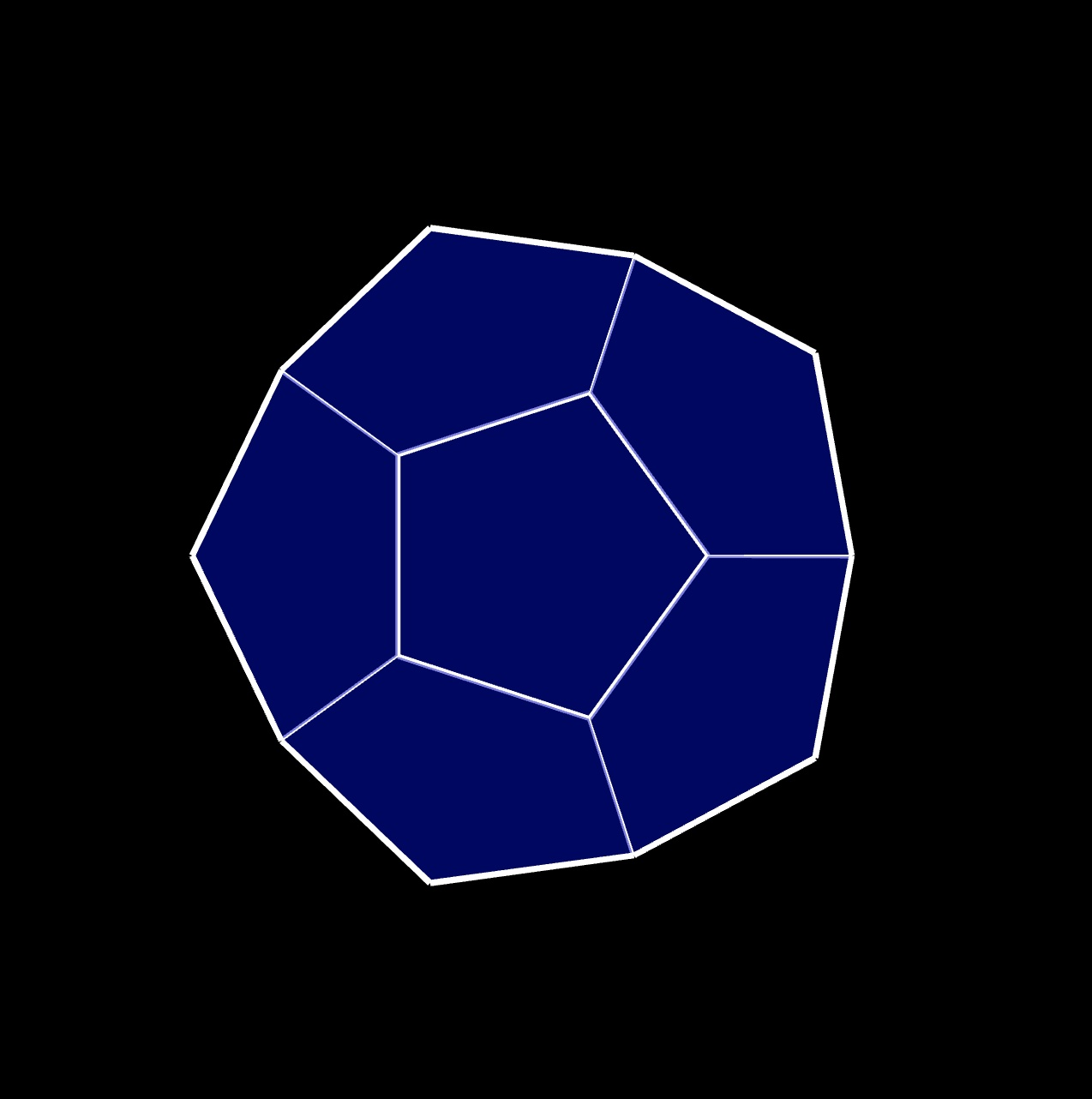the top ⬠ facing the bottom ⬠ :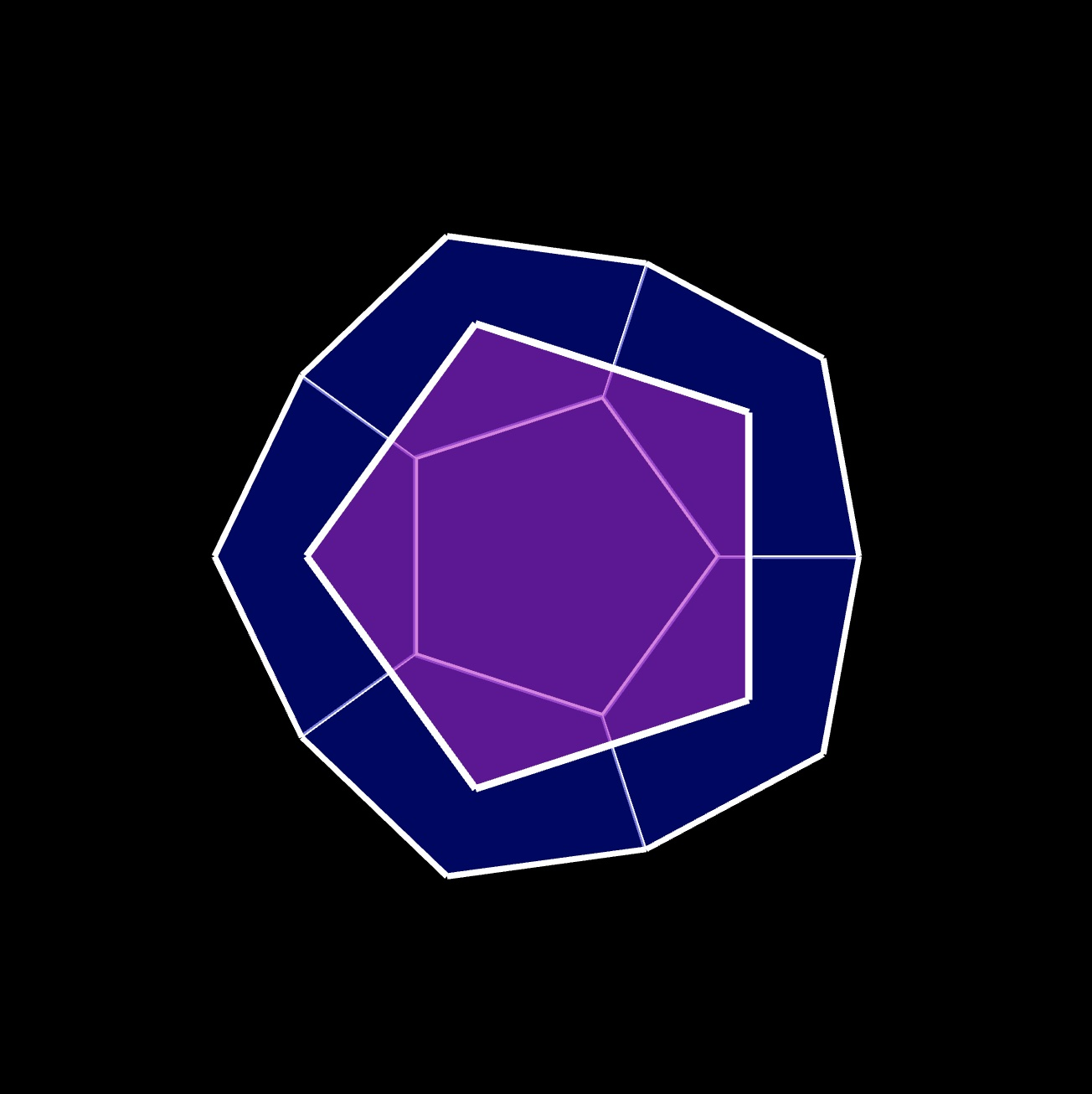the 1st ⬠ adjacent to the top ⬠ :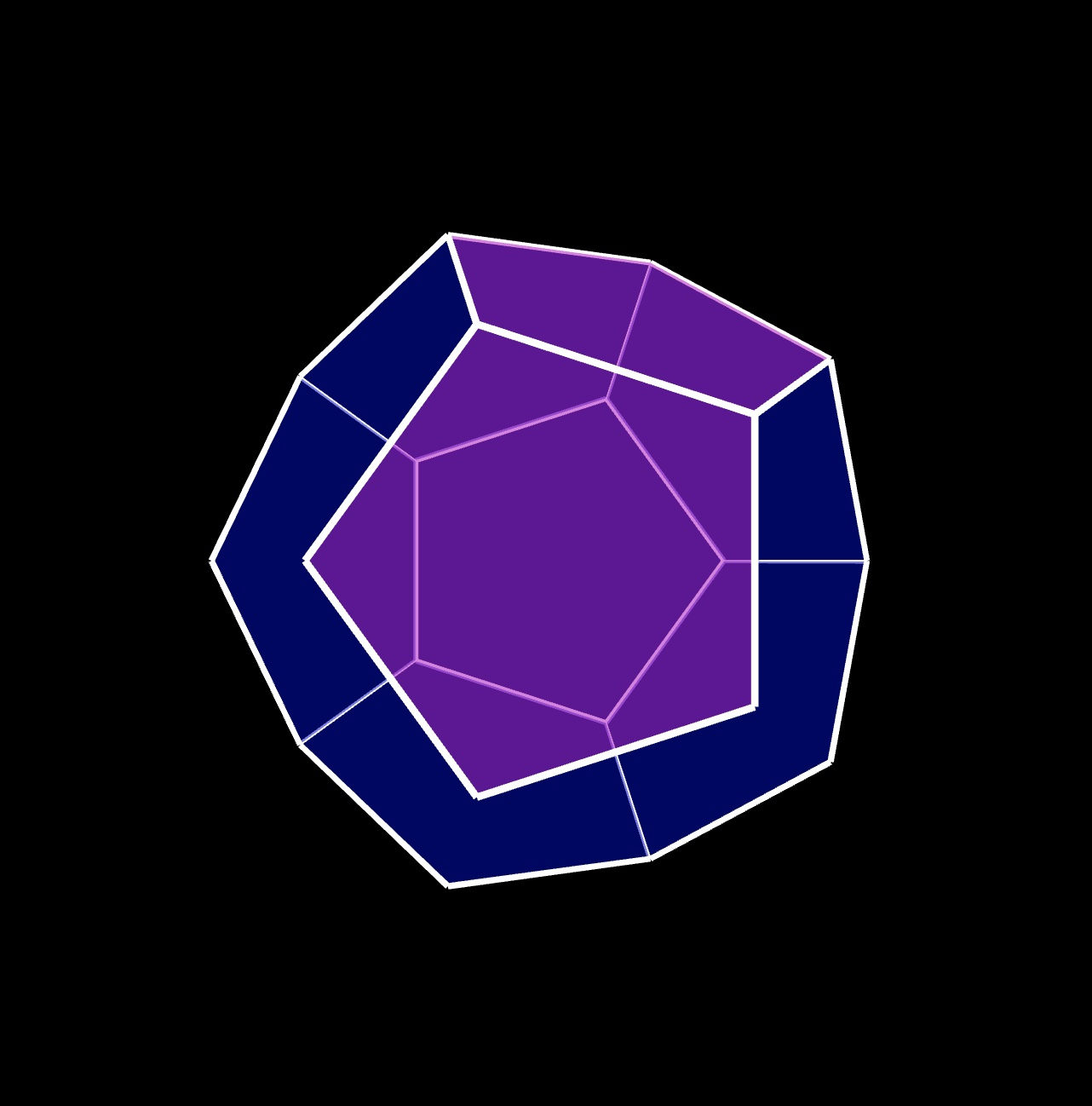the 2nd ⬠ adjacent to the top ⬠ :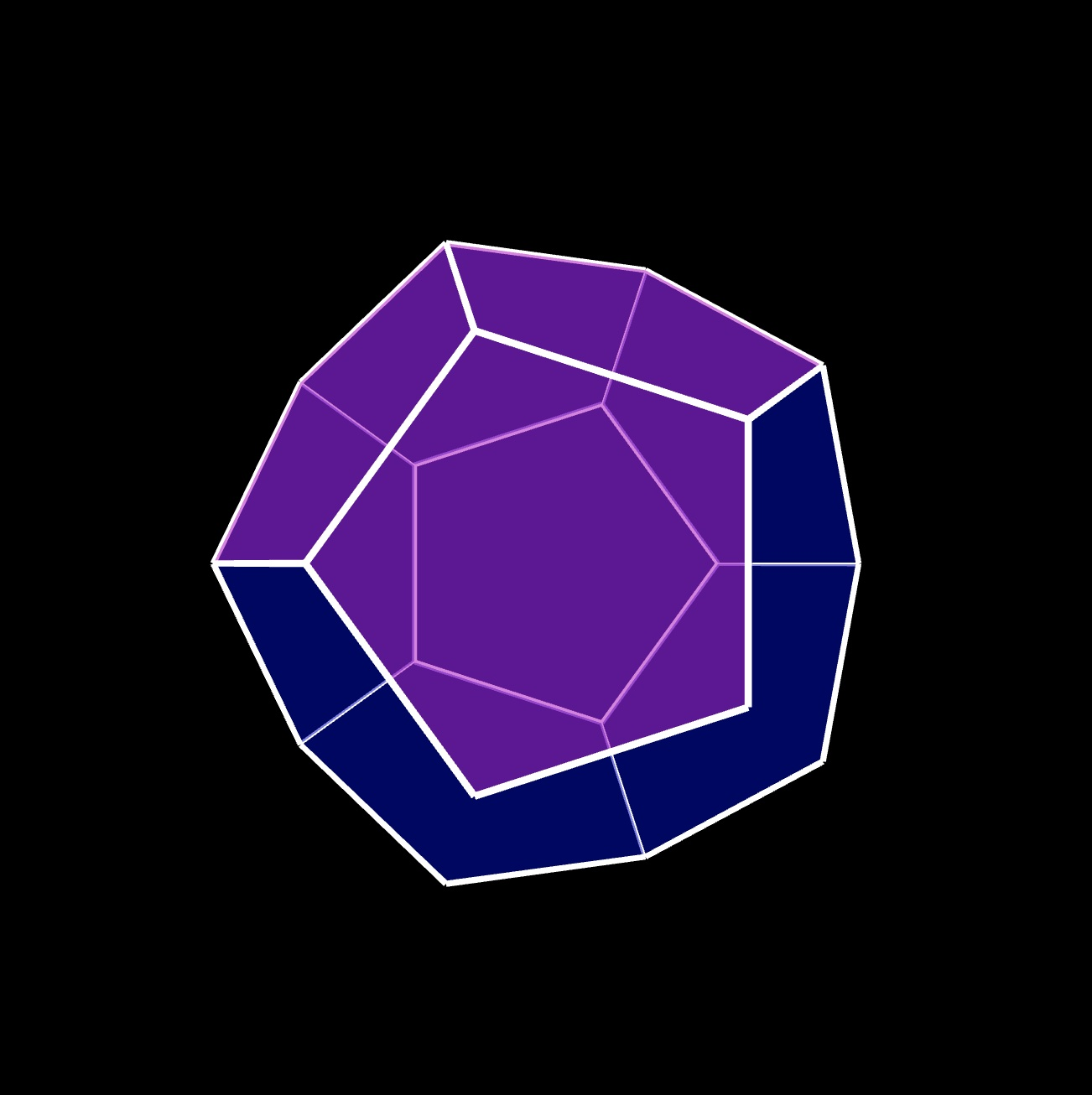the 3rd ⬠ adjacent to the top ⬠ :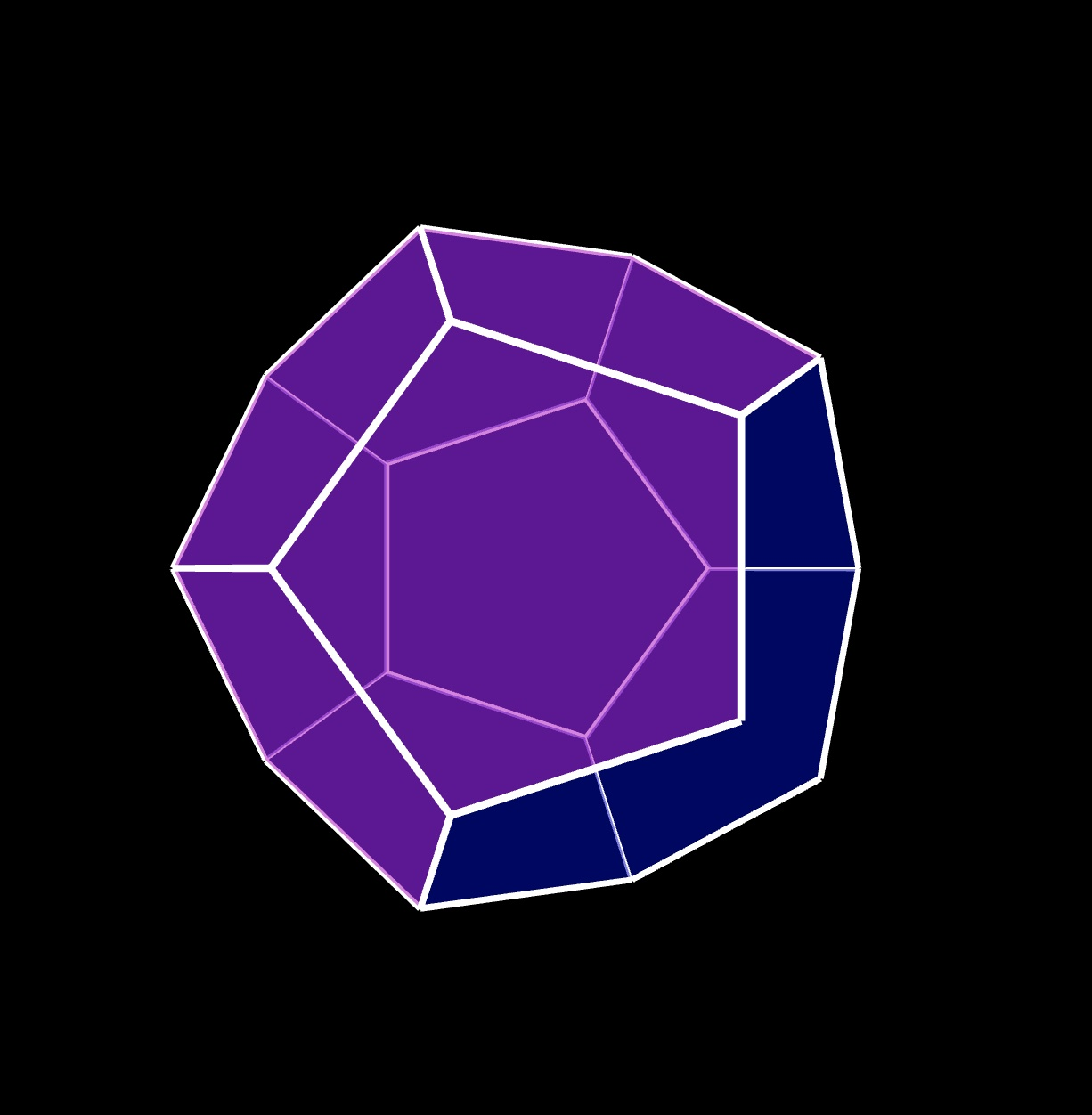the 4th ⬠ adjacent to the top ⬠ :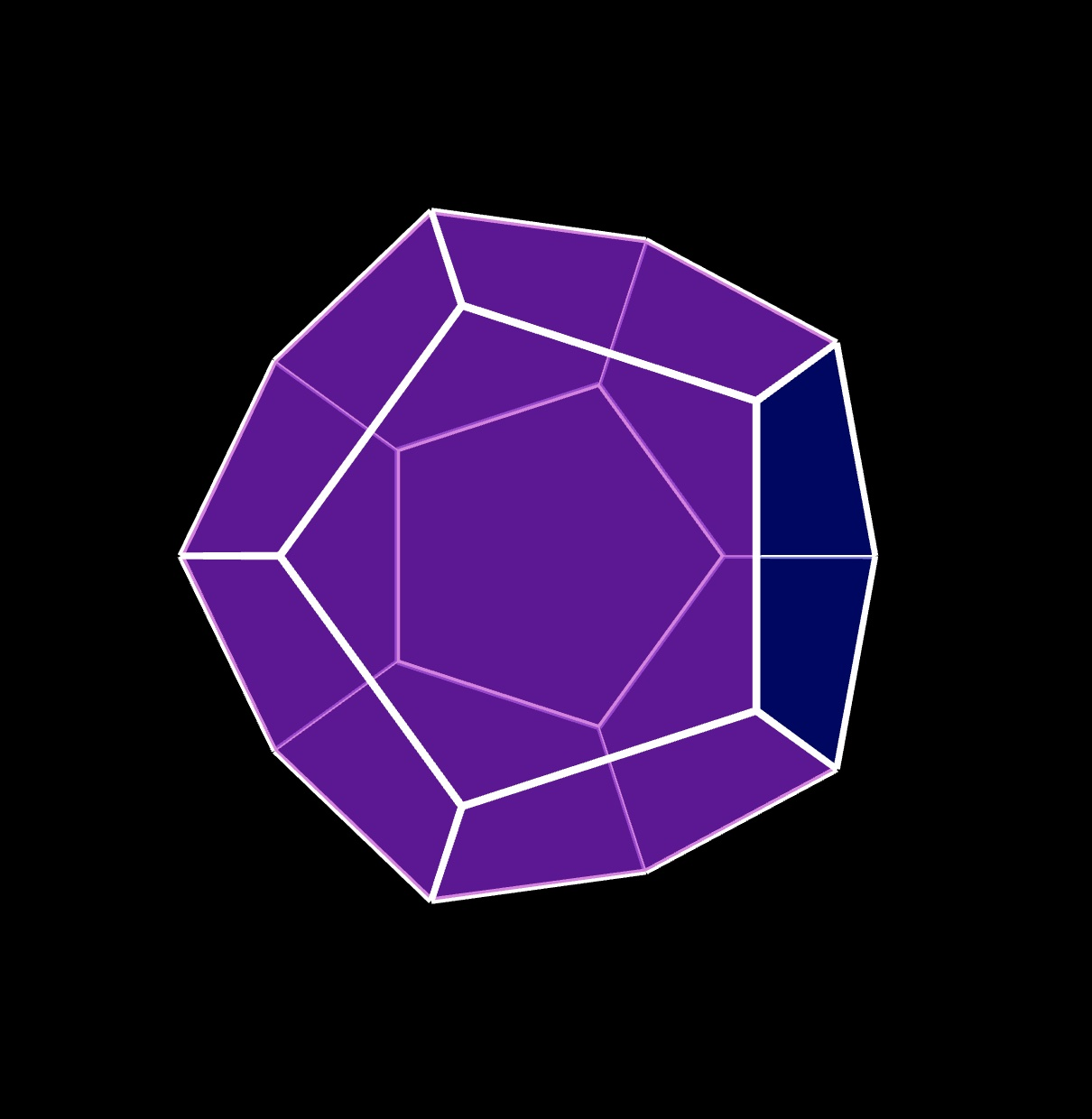``````

let ang = 0;
let r = 100;
let s,t,w,h;

function setup() {
createCanvas(960, 960, WEBGL);
stroke(255);
strokeWeight(3);
s = 2 * r * sin(PI/10);
t = r * cos(TWO_PI/10);
w = 2 * asin(sin(3*PI/10) /
sin(3*PI/5)); //116.7º
let GE = 2*r*sin(PI/5)*sin(PI/5);
let EH = r + t - GE;
h = sin(w) * (2 * EH + GE);
}

function penta(){
push();
translate(t,0,0);
beginShape();
for(let a = 0; a < TWO_PI;
a += TWO_PI/5) {
let x = r*cos(a);
let y = r*sin(a);
vertex(x, y);
}
endShape(CLOSE);
pop();
}

function half(){
push();
translate(-t,0,0);
penta();
pop();

push();
for(let i=0;i<5;i++){
rotateZ(TWO_PI/5);
push();
translate(-t,0,0);
rotateY(-w);
penta();
pop();
}
pop();
}

function draw() {
ang += 0.01;
background(0);
rotateY(ang);
rotateX(ang*0.5);
rotateZ(ang*0.2);

push();
fill(0,0,200,130);
half();
pop();

push();
fill(200,0,200,130);
translate(0,0,h);
rotateY(PI);
half();
pop();
}

``````

ch 2.2 The Regular Polyhedra p22 of